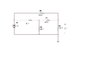• shaiqbashir

#### shaiqbashir

Hi Guys!

YOU HAVE BEEN GIVEN THAT THE VOLTAGE ACROSS THE RIGHT MOST 2 OHM RESISTOR IS 3V, AND NOW YOU HAVE TO FIND THE RESISTANCE "R" AND THE EQUIVALENT RESISTANCE OF THIS CIRCUIT.

please help me as soon as possible, i have to submit my assignment, solved all the questions already but this question is making troubles for me.

Plz help me

#### Attachments

•P1.36(c).JPG
8.1 KB · Views: 499

you could try a star-triangle transformation with the group R, R3, R4 it would simplify your circuit a bit

You can also solve it directly using Kirchoff's Law. You could, for instance, assume the currents are as shown in the pic, and solve for the 3 voltage loops and use the known drop across R5.

#### Attachments

And one knows the voltage drop across R2, which = V1 - 3 V (voltage across R5).

Also remember V = I * R or I = V/R.

I see nothing but two ohm resistors. If so then even if the unknown is infinite you would still not get as low as three volts for the output (across the rightmost resistor). So I would question the problem.
If you want two volts across the unknown then the problem is solvable.

The key is to redraw the circuit... follow the current path from one end of the power source, creating a parallel branch every time the path splits.

You should end up with a hybrid series-parallel circuit. If it gets too confusing, try drawing from the other direction.

Once you have redrawn the circuit, simplify by replacing the parallel sections with a single-resistance equivalent and resolve...

It takes a little time, but I usually solve for everything first, then go to the questions to answer.

shaiqbashir said:
Hi Guys!

YOU HAVE BEEN GIVEN THAT THE VOLTAGE ACROSS THE RIGHT MOST 2 OHM RESISTOR IS 3V, AND NOW YOU HAVE TO FIND THE RESISTANCE "R" AND THE EQUIVALENT RESISTANCE OF THIS CIRCUIT.

please help me as soon as possible, i have to submit my assignment, solved all the questions already but this question is making troubles for me.

Plz help me

This circuit cannot be simplified using the normal parallel/series rules. The first parallel branching resistor sticks in between two series resistors of yet another parallel branch making it loop back on itself.

You must use nodal analysis (particularly on the common node of R3, R4 and the unknown R). If you don't know what this is, solving this problem will be close to impossible. Secondly, because you cannot find the equivalent resistance using the parallel/series rule, you must use something called Thevenin's theorem, and find the thevenin resistance.

shaiqbashir said:
Hi Guys!

YOU HAVE BEEN GIVEN THAT THE VOLTAGE ACROSS THE RIGHT MOST 2 OHM RESISTOR IS 3V, AND NOW YOU HAVE TO FIND THE RESISTANCE "R" AND THE EQUIVALENT RESISTANCE OF THIS CIRCUIT.

please help me as soon as possible, i have to submit my assignment, solved all the questions already but this question is making troubles for me.

Plz help me

So did you get the answer? Using “Mesh Analysis” I arrived with 2 equations

-6i2 - 2Ri2 + (9/2)R + 3 = 0

2Ri2 - (9/2)R + 6 + 2i2 = 0

You can easily solve R or i2 using a calculator. But I’m not sure if my equations are right because I am so0o0ooo so0o0o0 sleEeEEeEEeeEpppyyyy :zzz: :zzz: :zzz: